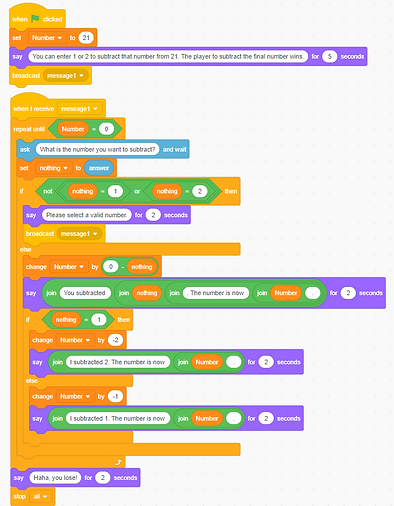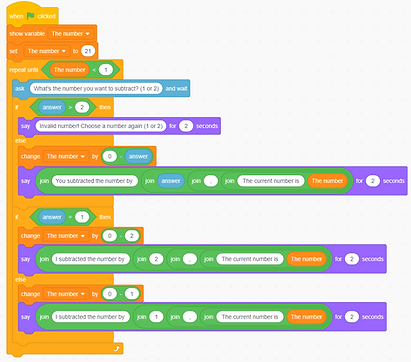top of page

# Count from 21 Game

Lesson Overview

​In this lesson, students will create a program of Count from 21 Game according to the game’s requirements, which encourages them to analyze the mathematical principles of the game and enhance the CT skills such as variable, conditional, iteration, decomposition and automation.

### Prior Knowledge

• Mathematics: operations

### Learning Objectives

1. To handle multiple conditional blocks simultaneously

2. To understand the relationship between conditional block and iteration block.

3. To acquire the mathematical principles and algorithms used in the game.

### Mathematics & CT Outcomes

• Mathematics: algorithms

• CT Skills: variable; conditional; iteration; decomposition (to break down the game problem into smaller programs); automation

### Teaching Resources

The Desmos Activity used in this Task.

## Lesson Details

In the following activity, you are going to develop a program called “Master of Count from 21” with your partner. The program is a one-player program which a player will play Count from 21 with computer. Your job is to make sure the computer wins all the time!

### Task 3: Create a Count from 21 game!

#### Program Requirement:

1. The computer must win the game.

2a. The computer will inform the player of the updated number.

2b. The computer will tell the player how many it subtracted and the updated number.

3. What should the program do if the player inputs an invalid number (e.g., 3)?

#### Students' Work

In this activity, students need to understand the rules and the mathematical principles of the game, i.e., the person who wants to win is not the initiator of each round, and the sum of the numbers subtracted from each round is kept at 3. The game requires students to examine the numbers input by the player into two categories: valid numbers (Number = 1 or Number = 2) and invalid numbers (Number ≠ 1 and Number ≠ 2). They need to process these different numbers differently by setting conditional blocks.In this work, the student applied [repeat until] and multiple [if...then...else...] blocks, and was able to nest the different conditional blocks appropriately. This demonstrates an understanding of the relationship between the different conditional and iteration blocks. First, the starting value of the game is determined by setting Number to 21. It is then made clear that the game stops when the number is reduced to 0, so that the iteration stops with the condition [repeat until Number = 0].

It is worth noting the way in which the input numbers are judged and handled in this work:

(1) If the number input is 1 or 2, it will execute “change Number by 0 – nothing”, and the computer will perform the next round of operations “if nothing = 1 then change Number by -2, else change Number by -1.”

(2) If the number input is not 1 or 2, it is considered invalid and the player will be reminded to input again (if not [nothing = 1] or [nothing = 2] then...). The conditionals block [if...then...] and operator [not...or...] are used in this case.Different from the previous work, this work uses the [forever] iteration block, and is set to stop when Number = 0. In addition, the [if…then…else…] block is used to examine valid and invalid numbers by setting answer=1, answer=2 and else in three different cases.

#### Possible Challenges and Misconceptions

1. Difficulty in using multiple [if... then... else...] blocks.

2. Wrong settings for judgment conditions.This work contains two [if...then...else...] blocks, which both have problems.

1. The first [if...then...else...] block can only examine the input numbers in two cases: “answer > 2” and “answer ≤ 2.” This cannot accurately restrict the input numbers to 1 and 2. Therefore, the judgment conditions need to be changed to “answer = 1 or answer = 2” and “answer ≠ 1 and answer ≠ 2”.

2. The second [if...then...else...] block is also only divided into two cases: answers = 1 and answers ≠ 1. This causes the else part of the loop [else change The number by 0 - 1] to be executed regardless of whether the number entered is a valid number (i.e., 1 and 2).